DensityBy Wikipedia,
the free encyclopedia,

http://en.wikipedia.org/wiki/Density

The density of a material is defined as its mass per unit volume. The symbol of density is ρ (the Greek letter rho).

## Formula

Mathematically: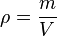$\rho = \frac{m}{V} \,$

where:

ρ is the density,
m is the mass,
V is the volume.

Different materials usually have different densities, so density is an important concept regarding buoyancy, metal purity and packaging.

In some cases density is expressed as the dimensionless quantities specific gravity (SG) or relative density (RD), in which case it is expressed in multiples of the density of some other standard material, usually water or air/gas.

## History

In a well-known common story, Archimedes was given the task of determining whether King Hiero's goldsmith was embezzling gold during the manufacture of a wreath dedicated to the gods and replacing it with another, cheaper alloy.

Archimedes knew that the irregularly shaped wreath could be crushed into a cube whose volume could be calculated easily and compared with the weight; but the king did not approve of this.

Baffled, Archimedes took a relaxing immersion bath and observed from the rise of the warm water upon entering that he could calculate the volume of the gold crown through the displacement of the water. Allegedly, upon this discovery, he went running naked through the streets shouting, "Eureka! Eureka!" (Greek "I found it"). As a result, the term "eureka" entered common parlance and is used today to indicate a moment of enlightenment.

This story first appeared in written form in Vitruvius' books of architecture, two centuries after it supposedly took place. Some scholars have doubted the accuracy of this tale, saying among other things that the method would have required precise measurements that would have been difficult to make at the time.

## Measurement of density

For a homogeneous object, the mass divided by the volume gives the density. The mass is normally measured with an appropriate scale or balance; the volume may be measured directly (from the geometry of the object) or by the displacement of a fluid. Hydrostatic weighing is a method that combines these two.

If the body is not homogeneous or heterogeneous, the density is a function of the coordinates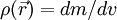$\rho(\vec{r})=dm/dv$, where dv is elementary volume with coordinates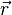$\vec{r}$. The mass of the body then can be expressed as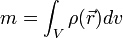$m = \int_V \rho(\vec{r})dv$,

where the integration is over the volume of the body V.

A very common instrument for the direct measurement of the density of a liquid is the hydrometer, which measures the volume displaced by an object of known mass. A common laboratory device for measuring fluid density is a pycnometer; a related device for measuring the absolute density of a solid is a gas pycnometer. Another instrument used to determine the density of a liquid or a gas is the digital density meter - based on the oscillating U-tube principle.

The density of a solid material can be ambiguous, depending on exactly how its volume is defined, and this may cause confusion in measurement. A common example is sand: if gently filled into a container, the density will be low; when the same sand is compacted into the same container, it will occupy less volume and consequently exhibit a greater density. This is because sand, like all powders and granular solids contains a lot of air space in between individual grains; this overall density is called the bulk density, which differs significantly from the density of an individual grain of sand.

## Common units

The SI unit for density is:

Metric units outside the SI

• kilograms per litre (kg/L). At 4 °C, water has a density of 1.000 kg/L, making this a convenient unit at about the room temperature,
• kilograms per cubic decimeter (kg/dm³),
• grams per millilitre (g/mL),
• grams per cubic centimeter (g/cc or g/cm³).

These are all numerically equivalent to kg/L (1 kg/L = 1 kg/dm³ = 1 g/cm³ = 1 g/mL).

In U.S. customary units or Imperial units, the units of density include:

## Changes of density

In general density can be changed by changing either the pressure or the temperature. Increasing the pressure will always increase the density of a material. Increasing the temperature generally decreases the density, but there are notable exceptions to this generalisation. For example, the density of water increases between its melting point at 0 °C and 4 °C and similar behaviour is observed in silicon at low temperatures.

The effect of pressure and temperature on the densities of liquids and solids is small so that a typical compressibility for a liquid or solid is 10 bar (1 bar=0.1 MPa) and a typical thermal expansivity is 10 K.

In contrast, the density of gases is strongly affected by pressure. Boyle's law says that the density of an ideal gas is given by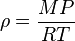$\rho = \frac {MP}{RT} \,$

where R is the universal gas constant, P is the pressure, M the molar mass, and T the absolute temperature.

This means that a gas at 300 K and 1 bar will have its density doubled by increasing the pressure to 2 bar or by reducing the temperature to 150 K.

Osmium is the densest known substance at standard conditions for temperature and pressure.

## Density of water

Temp (°C) Density (kg/m3)
100 958.4
80 971.8
60 983.2
40 992.2
30 995.6502
25 997.0479
22 997.7735
20 998.2071
15 999.1026
10 999.7026
4 999.9720
0 999.8395
−10 998.117
−20 993.547
−30 983.854
The density of water in kilograms per cubic meter (SI unit)
at various temperatures in degrees Celsius.
The values below 0 °C refer to supercooled water.

## Density of air

T in °C ρ in kg/m3 (at 1 atm)
–25 1.423
–20 1.395
–15 1.368
–10 1.342
–5 1.316
0 1.293
5 1.269
10 1.247
15 1.225
20 1.204
25 1.184
30 1.164
35 1.146

## Density of solutions

The density of a solution is the sum of the mass (massic) concentrations of the components of that solution.
Mass (massic) concentration of a given component ρi in a solution can be called partial density of that component.

## Density of composite material

ASTM specification D792-00 describes the steps to measure the density of a composite material.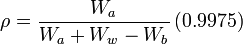$\rho = \frac{W_a}{W_a + W_w - W_b} \left (0.9975 \right ) \,$

where:

ρ is the density of the composite material, in g/cm

and

Wa is the weight of the specimen when hung in the air
Ww is the weight of the partly immersed wire holding the specimen
Wb is the weight of the specimen when immersed fully in distilled water, along with the partly immersed wire holding the specimen
0.9975 is the density in g/cm of the distilled water at 23°C

## Densities of various materials

Material ρ in kg/m3 Notes
Interstellar medium 10-25 − 10-15 Assuming 90% H, 10% He; variable T
Earth's atmosphere 1.2 At sea level
Aerogel 1 − 2
Styrofoam 30 − 120 From
Cork 220 − 260 From
Water 1000 At STP
Plastics 850 − 1400 For polypropylene and PETE/PVC
The Earth 5515.3 Mean density
Copper 8920 − 8960 Near room temperature
Tungsten 19250 Near room temperature
Gold 19300 Near room temperature
The Inner Core of the Earth ~13000 As listed in Earth
Uranium 19100 Near room temperature
Iridium 22500 Near room temperature
Osmium 22610 Near room temperature
The core of the Sun ~150000
White dwarf star 1 × 109
Atomic nuclei 2.3 × 1017  Does not depend strongly on size of nucleus
Neutron star 8.4 × 1016 — 1 × 1018
Black hole 4 × 1017 Mean density inside the Schwarzschild radius of an earth-mass black hole (theoretical)

Published in July 2009.